Next: Rotating Disk Up: Physical Background Previous: Jeans Instability   Contents

# Gravitational Instability of Thin DiskDisks are common in the Universe. Spiral and barred spiral galaxies have disks where stars are formed. In more small scale, gas and dust disks are often found around protostars. Moreover, such a disk may become a proto-planetary disk. It is valuable to study how the self-gravity works in such thin structures. Here, we assume a thin disk extending in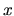- and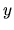-directions whose surface density is equal to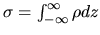, in other word the density is written using the Dirac's delta function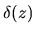as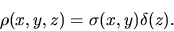(2.55)

Integrating along the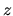-direction basic equations (2.45), (2.46), and (2.47), the linearized basic equations for the thin disk are as follows: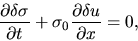(2.56)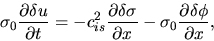(2.57)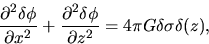(2.58)

where we assumed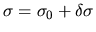,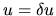,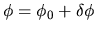and took the first order terms (those contain only one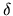).

Outside the disk, the rhs of equation (2.58) is equal to zero. It reduces to the Laplace equation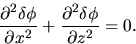(2.59)

Taking a plane wave of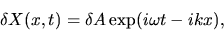(2.60)

equation (2.59) is reduced to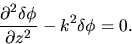(2.61)

This has a solution which does not diverge at the infinity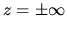as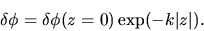(2.62)

On the other hand, integrating equation (2.58) from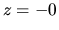to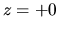or in other word, applying the Gauss' theorem to the region containing the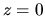surface, it is shown that the gravity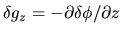has a jump crossing thesurface as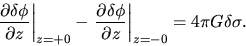(2.63)

Equations (2.62) and (2.63) lead a final form of the potential as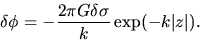(2.64)

Putting this to equations (2.57), and using equations (2.56) and (2.57), we obtain the dispersion relation for the gravitational instability in a thin disk as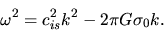(2.65)

This reduces to the dispersion relation of the sound wave for the short wave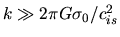. While for a longer wave than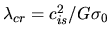, an exponential growth of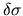is expected. The dispersion relation is shown in Fig.2.2.

SubsectionsNext: Rotating Disk Up: Physical Background Previous: Jeans Instability   Contents
Kohji Tomisaka 2007-07-08